### Exterior angle is sum of interior opposite angles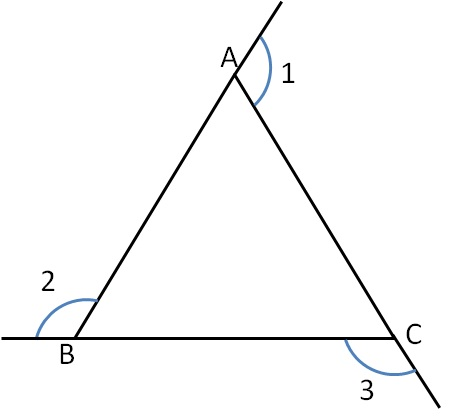For ∆ABC

∠1 = ∠ABC + ∠ACB

∠2 = ∠BAC + ∠ACB

∠3 = ∠BAC + ∠ABC

Let’s solve some questions

### Proof of exterior angle property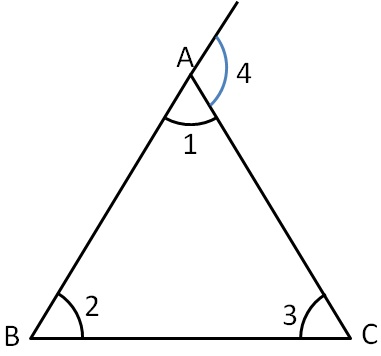In ∆ABC,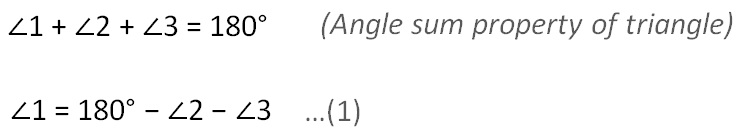Also,

∠1 + ∠4 = 180°           (Linear Pair)

(180° − ∠2 − ∠3) + ∠4 = 180°     (From (1 )

∠4 = 180° − 180° + ∠2 + ∠3

∠4 = 0° + ∠2 + ∠3

∠4 = ∠2 + ∠3

∴ Exterior angle is equal to sum of interior opposite angles

### Find exterior angle ∠ 1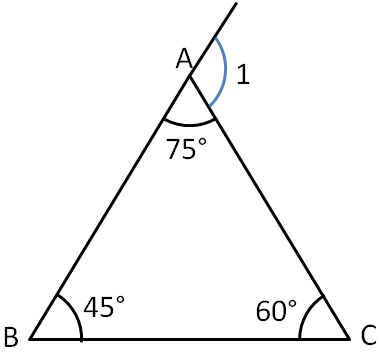Here,

∠1 = ∠B + ∠C     ( Exterior angle property)

∠1 = 45°  + 60°

∠1 = 105°

### Find exterior angle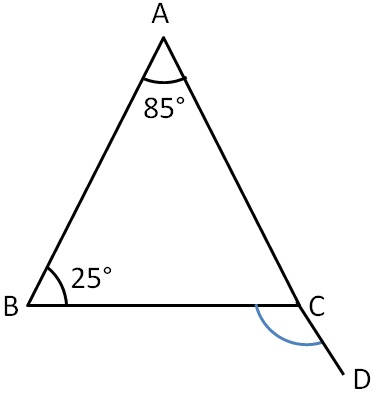In ∆ABC,

∠BCD = ∠CAB + ∠ABC        (Exterior angle property)

∠BCD = 85° + 25°

∠BCD = 110°

### Find exterior angle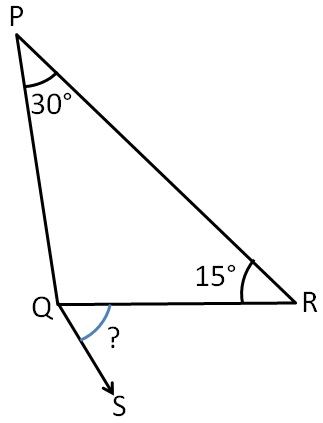In ∆PQR,

∠SQR = ∠P + ∠R        (Exterior angle property)

∠SQR = 30° + 15°

∠SQR = 45°

### Find exterior angle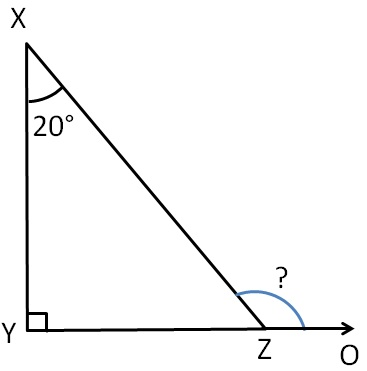In ∆XYZ,

∠XZO = ∠X + ∠Y           (Exterior angle property)

∠XZO = 20° + 90°

∠XZO = 110°

### Find ∠ACB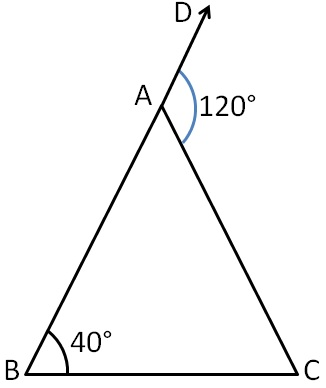In ∆ABC,

∠DAC = ∠B + ∠C     (Exterior angle property)

120° = 40° + ∠C

120° − 40° = ∠C

80° = ∠C

∠C = 80°

i.e. ∠ACB = 80°

### Find ∠PQR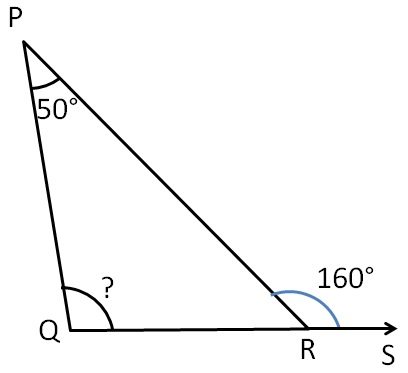In ∆PQR,

∠PRS = ∠PQR + ∠QPR        (Exterior angle property)

160° = ∠PQR  + 50°

160° − 50° = ∠PQR

110° = ∠PQR

∠PQR = 110°

### Find ∠XZY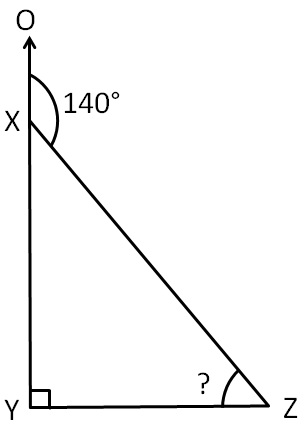In ∆XYZ,

∠OXZ = ∠XZY + ∠XYZ        (Exterior angle property)

140° = ∠XZY  + 90°

140° − 90° = ∠XZY

50° = ∠XZY

∠XZY = 50°

Subscribe to our Youtube Channel - https://you.tube/teachoo

1. Chapter 6 Class 7 Triangle and its Properties
2. Concept wise
3. Exterior angles of a triangle

Exterior angles of a triangle